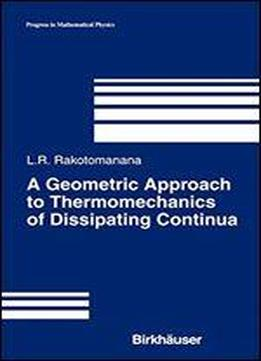# A Geometric Approach To Thermomechanics Of Dissipating Continua by Lalao Rakotomanana / 2003 / English / PDF

Across the centuries, the development and growth of mathematical concepts have been strongly stimulated by the needs of mechanics. Vector algebra was developed to describe the equilibrium of force systems and originated from Stevin's experiments (1548-1620). Vector analysis was then introduced to study velocity fields and force fields. Classical dynamics required the differential calculus developed by Newton (1687). Nevertheless, the concept of particle acceleration was the starting point for introducing a structured spacetime. Instantaneous velocity involved the set of particle positions in space. Vector algebra theory was not sufficient to compare the different velocities of a particle in the course of time. There was a need to (parallel) transport these velocities at a single point before any vector algebraic operation. The appropriate mathematical structure for this transport was the connection. I The Euclidean connection derived from the metric tensor of the referential body was the only connection used in mechanics for over two centuries. Then, major steps in the evolution of spacetime concepts were made by Einstein in 1905 (special relativity) and 1915 (general relativity) by using Riemannian connection. Slightly later, nonrelativistic spacetime which includes the main features of general relativity I It took about one and a half centuries for connection theory to be accepted as an independent theory in mathematics. Major steps for the connection concept are attributed to a series of findings: Riemann 1854, Christoffel 1869, Ricci 1888, Levi-Civita 1917, WeyJ 1918, Cartan 1923, Eshermann 1950.

views: 482